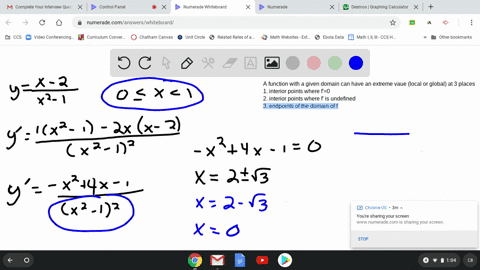Enroll in one of our FREE online STEM bootcamps. Join today and start acing your classes!View Bootcamps### a. Identify the function's local extreme values i…

09:10Georgia Southern University
Problem 50

# a. Identify the function's local extreme values in the given domain, and say where they occur.b. Which of the extreme values, if any, are absolute?c. Support your findings with a graphing calculator or computer grapher.$$f(x)=\sqrt{x^{2}-2 x-3}, \quad 3 \leq x<\infty$$

## Discussion

You must be signed in to discuss.

## Video Transcript

So here we have affects is he square it of X squared minus two X minus three on the domain X between three and infinity. Let's go and take the derivative To find the critical points that's gonna be one over to square it X squared minus two X minus three and then apply the chain rule. So on top will have two X minus two. And so when does f prime Nickel zero plus, when the numerator is euros, seven x is one, but notice that X is one is not into domain. So we'LL throw that one away And when his f prime undefined Well, that's gonna happen when the denominators zero. So, in other words, when x squared minus two x prentiss three zero. Okay, if you factor this this is X minus three times X plus one call zero. So you have excess three or exits, negative one. It can notice. The negative one is not the domain. It's our only critical point is a three killer. So then we only all we need to know is whether or not this function is increasing or decreasing after three well free plug in, say, four. We're going to get eight minus twos. That's positive. F crime is going to be positive, so this function is increasing after three. So that means we have a local minimum. So again, this is going to be positive honor to me. So we have a local men whenever the function starts at X equals three, three of and the value is f of three, which is here, and this will also be an absolute mess because it's going to increase after. For ex greater than three, it's going to increase off to infinity, so there's no absolute maximum.